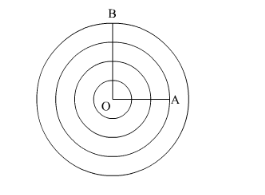# The radius of a circle is 20 cm.Question:

The radius of a circle is 20 cm. It is divided into four parts of equal area by drawing three concentric circles inside it. Then, the radius of the largest of three concentric circles drawn is

(a) $10 \sqrt{5} \mathrm{~cm}$

(b) $10 \sqrt{3} \mathrm{~cm}$

(c) $10 \sqrt{5} \mathrm{~cm}$

(d) $10 \sqrt{2} \mathrm{~cm}$

Solution:

The circle can be divided into four parts of equal area by drawing three concentric circles inside it as,It is given that OB = 20 cm. Let OA = x.

Since the circle is divided into four parts of equal area by the three concentric circles, we have,

Area of the fourth region $=\frac{1}{4} \times$ Area of the given circle

$\pi \times\left(20^{2}-x^{2}\right)=\frac{1}{4} \times \pi \times 20^{2}$

$400-x^{2}=100$

$x^{2}=300$

$x=10 \sqrt{3} \mathrm{~cm}$

Therefore, the correct answer is (b).